# Failure of Clairaut's theorem where both mixed partials are defined but not equal

## Statement

### For a function of two variables at a point

It is possible to have a function$f$ of two variables$x,y$ and a point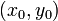$(x_0,y_0)$ in the domain of$f$ such that both the second-order mixed partial derivatives of$f$ exist at$(x_0,y_0)$, i.e., both the numbers$f_{xy}(x_0,y_0)$ and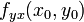$f_{yx}(x_0,y_0)$ exist, but they are not equal.

### For a function of two variables overall

It is possible to have a function$f$ of two variables$x,y$ such that both the second-order mixed partial derivatives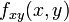$f_{xy}(x,y)$ and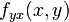$f_{yx}(x,y)$ exist everywhere on$\R^2$ but they are not equal as functions, i.e., there exists a point where the values of the second-order mixed partial derivatives are not equal.

## Proof

### Example

Consider the function:$f(x,y) := \left\lbrace \begin{array}{rl} \frac{xy(x^2 - y^2)}{x^2 + y^2}, & (x,y) \ne (0,0) \\ 0, & (x,y) = (0,0) \\\end{array}\right.$

We do some computations:

Item Value Explanation$f_x(x,y)$ for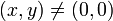$(x,y) \ne (0,0)$$\frac{(x^2 + y^2)(3x^2y - y^3) - 2x^2y(x^2 - y^2)}{(x^2 + y^2)^2} = \frac{x^4y + 4x^2y^3 - y^5}{(x^2 + y^2)^2}$ use the quotient rule for differentiation and simplify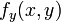$f_y(x,y)$ for$(x,y) \ne (0,0)$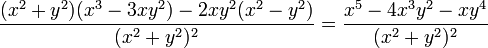$\frac{(x^2 + y^2)(x^3 - 3xy^2) - 2xy^2(x^2 - y^2)}{(x^2 + y^2)^2} = \frac{x^5 - 4x^3y^2 - xy^4}{(x^2 + y^2)^2}$ use the quotient rule for differentiation and simplify$f_x(0,y)$ for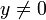$y \ne 0$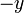$-y$ plug in$x = 0$ in the general expression for$f_x(x,y)$ and simplify.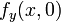$f_y(x,0)$ for$x \ne 0$$x$ plug in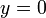$y = 0$ in the general expression for$f_y(x,y)$ and simplify.$f_x(0,0)$ 0 start with$\lim_{x \to 0} \frac{f(x,0) - f(0,0)}{x}$ and simplify, noting that the numerator is identically zero$f_y(0,0)$ 0 start with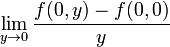$\lim_{y \to 0} \frac{f(0,y) - f(0,0)}{y}$ and simplify, noting that the numerator is identically zero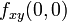$f_{xy}(0,0)$ -1 start with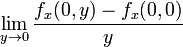$\lim_{y \to 0} \frac{f_x(0,y) - f_x(0,0)}{y}$ and simplify using the expressions obtained above for$f_x(0,y)$ and$f_x(0,0)$$f_{yx}(0,0)$ 1 start with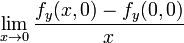$\lim_{x \to 0} \frac{f_y(x,0) - f_y(0,0)}{x}$ and simplify using the expressions obtained above for$f_y(x,0)$ and$f_y(0,0)$